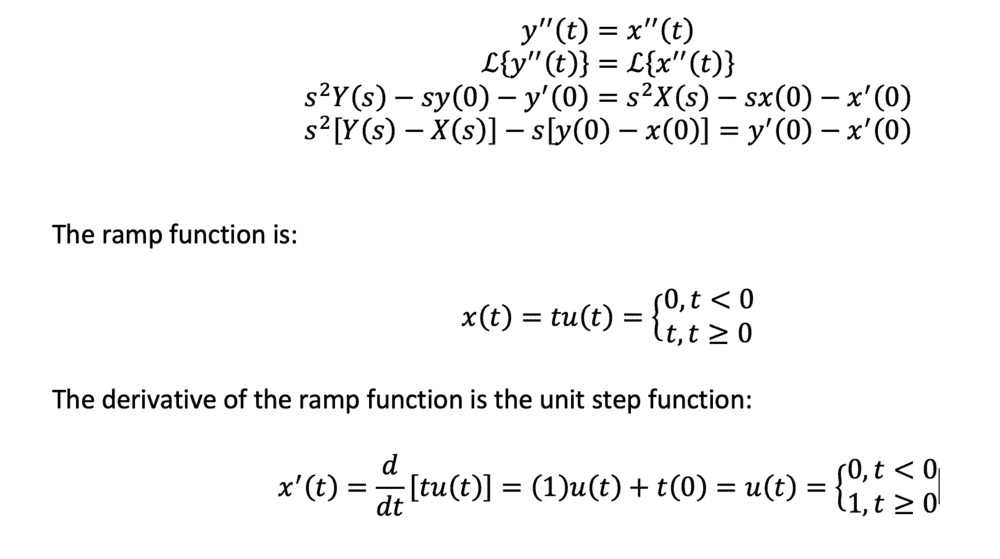# Laplace transform of an ODE with a non-smooth forcing function

StretchySurface
Homework Statement:
How do I deal with non-smooth forcing functions if I want to solve the Laplace transform of an ode.
Relevant Equations:
See below
Suppose I'm solving
$$y''(t) = x''(t)$$ where $$x(t)$$ is the ramp function. Then, by taking the Laplace transform of both sides, I need to know $x'(0)$ which is discontinuous. What is the appropriate technique to use here?

Homework Helper
Check the screenshot I attached:•FactChecker
•